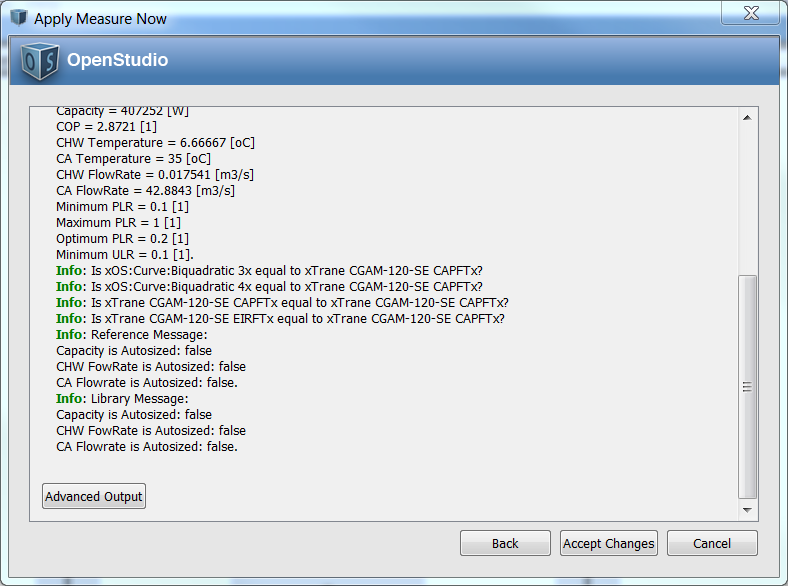Question-and-Answer Resource for the Building Energy Modeling Community
Get s tarted with the Help page

# replace, not clone, a curve?I was able to use an existing code snippet to create (or clone, if it already exists) a particular curve. However, if a curve of that name (say "Curve") already exists, then this will add another curve (say "Curve 1"), and each time this is called, there will be another (say "Curve 2", etc.) - the meaning of clone, I suppose.

 library_Factor2 = curve.get.to_ModelObject.get.clone(model).to_CurveBiquadratic.get 

Is there a way to replace (or first delete and then create) that curve, so that I will only ever have a curve named "Curve" in the model? Something like (hopefully it is that easy) the following, perhaps?

 library_Factor2 = curve.get.to_ModelObject.get.replace(model).to_CurveBiquadratic.get 

Where the replace function would either create or replace, not create or clone?

<<< EDIT <<<

OK, some progress, thanks. I did the following:

model_Curves = model.getCurveBiquadratics
model_Curves.each do |model_Curve|
runner.registerInfo("Is x#{model_Curve.name}x equal to x#{curve_name}x?")
if model_Curve.name == curve_name
runner.registerInfo("Yes, x#{model_Curve.name}x is equal to x#{curve_name}x!")
break
end
end


Unfortunately, it does not quite work, as the result in the image below shows.It seems to me that "Trane CGAM-120-SE CAPFT" should have been caught and entered the above if comparison, but it does not seem to? Also, should I be able to enter the if comparison, I could then simply use

model_Curve.remove


Is that correct?

edit retag close merge delete

1

Try if model_Curve.name.to_s == curve_name.

model_Curve.name is returning an object, so if you're checking equality with a string it won't work. You can make it a string with to_s.

OK, so the following works, whereby model_Curve.name.get and model_Curve.name.to_s seem to give the same results.

    model_Curves = model.getCurveBiquadratics
model_Curves.each do |model_Curve|
runner.registerInfo("Is x#{model_Curve.name}x equal to x#{curve_name}x?")
if model_Curve.name.get == curve_name.get
runner.registerInfo("Yes, x#{model_Curve}x is equal to x#{curve.get}x!")
runner.registerInfo("Yes, x#{model_Curve.name}x is equal to x#{curve_name}x!")
model_Curve.remove
break
end
end


... unfortunately, the model_Curve.remove part does not work. I suppose I cannot just delete the curve without also deleting it in the object that references it. I tried to do that with model_chiller.setCoolingCapacityFunctionOfTemperature(), but it does not let me do that. I know I could reassign all the curve entries if the curvealready exists, but the remove/clone approach seems to require less programming.

I'm confused at what the ultimate aim is. Are you trying to add a new curve that might already exist in the model? Or add a curve that is named the same as an existing curve, but contains different data?

If you want to check if a curve named "Curve" exists in the model, you can test if model.getCurveByName("Curve").is_initialized returns true, and then not add an additional "Curve".

Sort by » oldest newest most voted

You could find your Curve by getting all the curves in the model and then finding the one named "Curve".

mycurves = model.getCurveBiquadratics
theCurve=[]
mycurves .each do |mcurve|
if mcurve.name.to_s == 'Curve'
theCurve = mcurve
break
end
end


Then you can use the methods listed here to change the Curve. Alternatively, you could stay with the boost code you used and once you done with the first curve delete it using .remove and rename the new one to whatever you need.

more

OK, so I am trying the following:

model_curve = model.getCurveByName("Curve")
if model_curve.is_initialized()
runner.registerInfo("Coefficient 1: #{model_curve.get.coefficient1Constant()}")
else
runner.registerInfo("Curve #{curve_name.get} is not yet initialized!")
end


The decision towards "already initialized" or "not yet initialized" is made correctly. However, it fails at trying to display coefficient1Constant(). Am I misusing the "get" function? What would fix this?

You need to change to :

model_curve = model.getCurveByName("Curve")
if model_curve.is_initialized()
runner.registerInfo("Coefficient 1: #{model_curve.get.coefficient1Constant()}")
else
runner.registerInfo("Curve #{model_curve.get.name.to_s} is not yet initialized!")
end


That is since model.getCurveByName returns boost object, then when you use the .get you translate it to curve object which then accept the normal methods (name etc.)

Yes, in the end, I was able to do initialization checks on these entities:

  model_curve_check = model.getCurveByName(curve_name.get)


And if these curves exist, I can do any work I want on them by doing the following:

if model_curve_check.is_initialized and library_curve_check.is_initialized then

library_coefficient1 = library_curve.coefficient1Constant()

if library_coefficient1 != "" then
model_curve.setCoefficient1Constant(library_coefficient1)
end

model_chiller.setCoolingCapacityFunctionOfTemperature(model_curve)
end


And if only the library curve exists, then I do the following:

if not model_curve_check.is_initialized and library_curve_check.is_initialized then

model_chiller.setCoolingCapacityFunctionOfTemperature(library_curve)
end


I even encapsulated this in its own function, one for biquadratic, one for quadratic.

This seems to work reasonably well now.

more